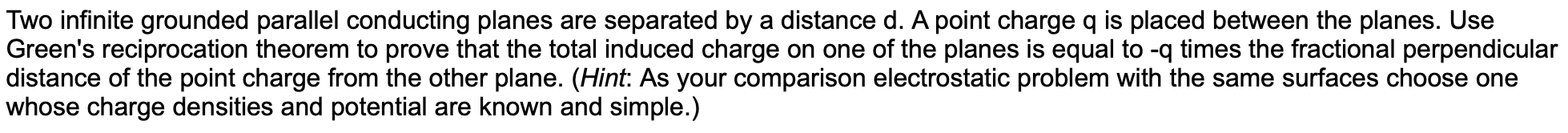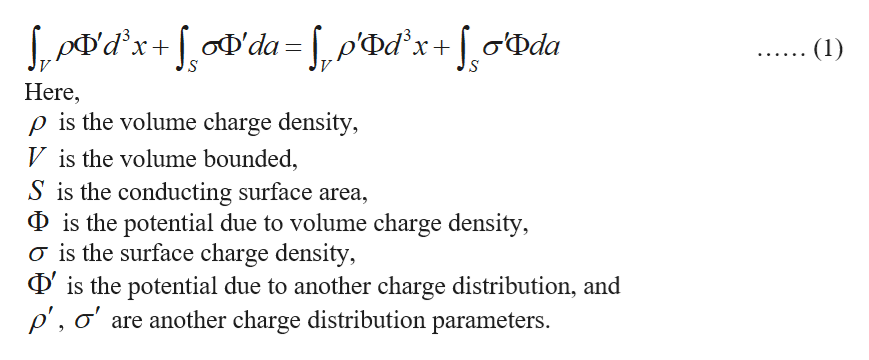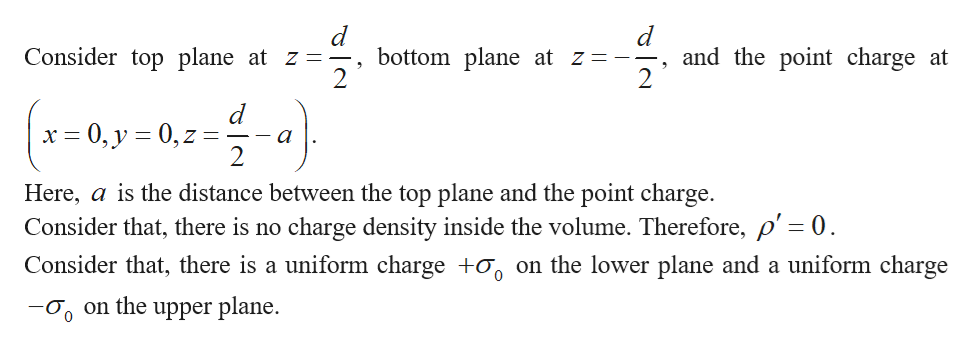Two infinite grounded parallel conducting planes are separated by a distance d. A point charge q is placed between the planes. UseGreen's reciprocation theorem to prove that the total induced charge on one of the planes is equal to -q times the fractional perpendiculardistance of the point charge from the other plane. (Hint: As your comparison electrostatic problem with the same surfaces choose one|whose charge densities and potential are known and simple.)

Question

3help_outlineImage TranscriptioncloseTwo infinite grounded parallel conducting planes are separated by a distance d. A point charge q is placed between the planes. Use Green's reciprocation theorem to prove that the total induced charge on one of the planes is equal to -q times the fractional perpendicular distance of the point charge from the other plane. (Hint: As your comparison electrostatic problem with the same surfaces choose one |whose charge densities and potential are known and simple.) fullscreen
Step 1

Write the Green’s reciprocation theorem as follows:help_outlineImage Transcriptionclosedx'da= , p«Dd°x + [ o{Dda (1) Here p is the volume charge density, V is the volume bounded, S is the conducting surface area Dis the potential due to volume charge density, o is the surface charge density, Dis the potential due to another charge distribution, and p', o' are another charge distribution parameters fullscreen
Step 2help_outlineImage Transcriptioncloseand the point charge at 2 Consider top plane at z = bottom plane at z = -- 2 (-0y-02-) d x 0, y 0, z a Here, a is the distance between the top plane and the point charge. Consider that, there is no charge density inside the volume. Therefore, p' = 0 Consider that, there is a uniform charge +o on the lower plane and a uniform charge on the upper plane. 0 fullscreen
Step 3

Use the symmetrical property, physical considerations, and write the electr...

Want to see the full answer?

See Solution

Want to see this answer and more?

Our solutions are written by experts, many with advanced degrees, and available 24/7

See Solution
Tagged in

Electrical Engineering